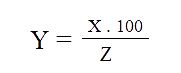Light Mode

# Percentage calculator

#### What is x % of y ? (Eg: 5% of 100 equal 5)

Formula:#### x is what % of y ? (Eg: 5 is 5% of 100 )

Formula:#### x is y% of what? (Eg: 5 is 1% of 500 )

Formula:Other Tools